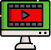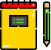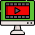VideosNotes

### Approximation

Logarithm - Quotient Rule
Since eln(x/y)=eln(x)−ln(y), we can conclude that the quotient rule for logarithms is ln(x/y)=ln(x)−ln(y)
Views: N/A
Video Duration: 4:47
On the Same TopicLOGARITHMS
Logarithm, the exponent or ...Logarithmic Equations
i. Revision of logarithm of...Anti-Logarithm
The anti-logarithm of a num...LOGARITHMS NUMBERS
Logarithm, the exponent or ...
Other Related Videos